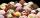# Effective and mean voltage

A voltage divider consisting of resistors R1 = 103000 Ω and R2 = 197000 Ω is connected to the ideal sine wave voltage source, R2 is connected to a voltmeter which measures the mean voltage and has an internal resistance R3 = 200300 Ω, the measured value is 109 V. Determine the effective source voltage.

Result

U1 =  246.625 V

#### Solution:Leave us a comment of example and its solution (i.e. if it is still somewhat unclear...):

Showing 0 comments:Be the first to comment!## Next similar examples:

1. 75th percentile (quartille Q3)Find 75th percentile for 30,42,42,46,46,46,50,50,54
2. Median and modusRadka made 50 throws with a dice. The table saw fit individual dice's wall frequency: Wall Number: 1 2 3 4 5 6 frequency: 8 7 5 11 6 13 Calculate the modus and median of the wall numbers that Radka fell.
3. ResistanceA resistor having an electrical resistance of 1.5 k ohms passes an electrical current of 0.1 A. Calculate what voltage is between the terminals of the resistor.
4. Theorem proveWe want to prove the sentense: If the natural number n is divisible by six, then n is divisible by three. From what assumption we started?
5. CalculationHow much is sum of square root of six and the square root of 225?
6. DiscriminantDetermine the discriminant of the equation: ?
7. Complex number coordinatesWhich coordinates show the location of -2+3i
8. Sequence 3Write the first 5 members of an arithmetic sequence: a4=-35, a11=-105.
9. EquationEquation ? has one root x1 = 8. Determine the coefficient b and the second root x2.
10. The confectioneryThe confectionery sold 5 kinds of ice cream. In how many ways can I buy 3 kinds if order of ice creams does not matter?
11. SeatsSeats in the sport hall are organized so that each subsequent row has five more seats. First has 10 seats. How many seats are: a) in the eighth row b) in the eighteenth row
12. Geometric progression 2There is geometric sequence with a1=5.7 and quotient q=-2.5. Calculate a17.
13. TrinityHow many different triads can be selected from the group 43 students?
14. Quadratic equationFind the roots of the quadratic equation: 3x2-4x + (-4) = 0.
15. SequenceBetween numbers 1 and 53 insert n members of the arithmetic sequence that its sum is 702.
16. RootsDetermine the quadratic equation absolute coefficient q, that the equation has a real double root and the root x calculate: ?
17. Linsys2Solve two equations with two unknowns: 400x+120y=147.2 350x+200y=144